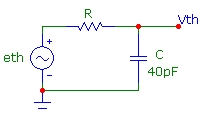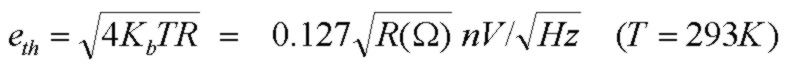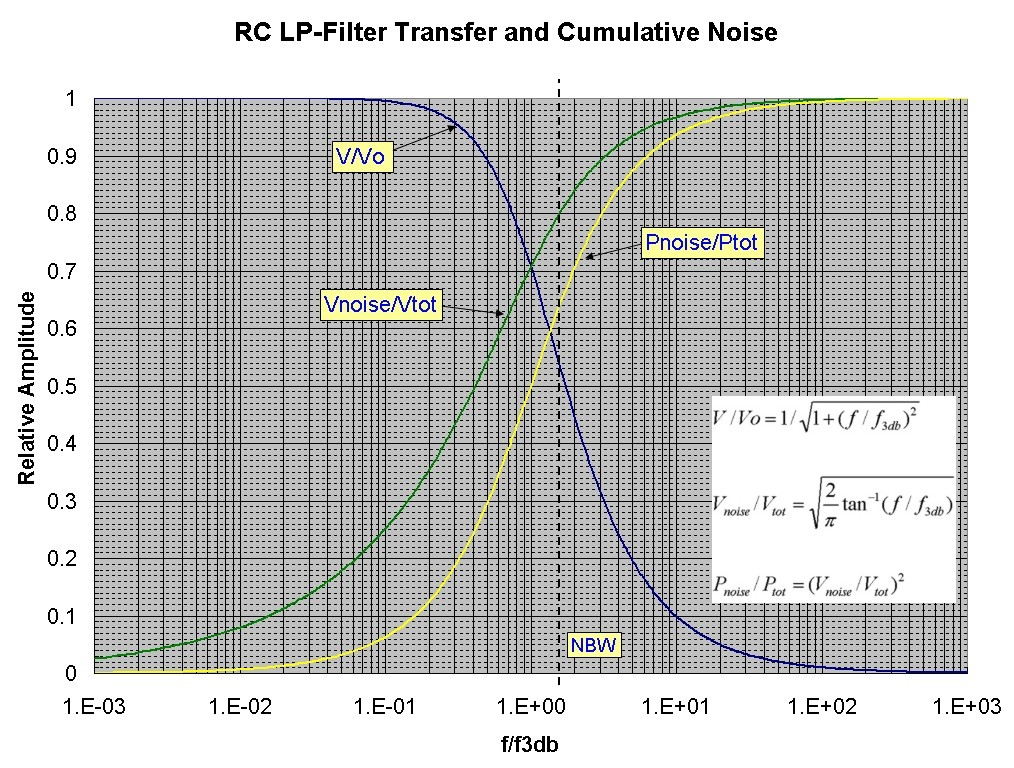# Thermal Noise

July 5, 2012The chart shows the cumulative and total thermal noise of an RC filter for C=40pF at T=293K . eth is the constant thermal noise voltage spectral density. The value of en is the intercept of the curves at f=1Hz per unit bandwidth. The curves are Vth(f), the cumulative voltage noise across C up to frequency f in μV units. Each curve corresponds to a different R value as indicated. Vo(C) is the asymptotic high-frequency value of the curves and is the total output thermal voltage across the capacitor integrated over all frequency. For this set of curves with C=40pF, Vo(C)=10 µV. The scale on the right indicates the Vo(C) value for other values of C. Graphically, the f3db bandwidth for a given curve is the intercept of that curve with a horizontal 7.07µV line. Graphically, the noise bandwidth, as defined below, is the intercept of the extended low-frequency part of the curve (with slope 1/2) with the horizontal Vo(C) line (10 µV for this set of curves). For more information on noise and noise-bandwidth, see Cumulative Thermal Noise:The chart below shows the voltage transfer function, cumulative noise voltage and cumulative noise power on a linear-log plot. The noise-bandwidth is shown as a dashed vertical line: